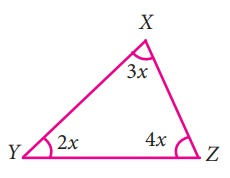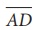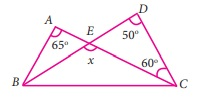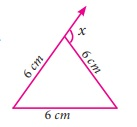Home | | Maths 7th Std | Exercise 4.1 (angles of triangle)

# Exercise 4.1 (angles of triangle)

Text Book Back Exercises Questions with Answers, Solution

Exercise 4.1

1. Can 30°, 60° and 90° be the angles of a triangle?

Solution:

Given angles 30°, 60°, 90°

Sum of the angles = 30°+ 60°+ 90° = 180°

The given angles can form a triangle.

2. Can you draw a triangle with 25°, 65° and 80° as angles?

Solution:

Given angles 25°, 65°, 80°

Sum of the angles = 25°+ 65°+ 80° = 170° ≠ 180°

The given angles can not form a triangle.

3. In each of the following triangles, find the value of x.Solution:

i) E + F + G = 180°80° + 55° + x =180°

135° + x =180°

x = 180° – 135° = 45°

x = 45°

ii) M + N + O = 180°x + 96° + 22° =180°

x + 118° =180°

x =180° – 118o = 62o

x = 62°

iii) X + Y + Z =180°29° + 90° + 2x + l = 180°

120° + 2x =180°

2x =180° – 120o = 60o

x = 30°

iv) L + J + K = 180°3x + x +112° = 180°

4x + 112° = 180°

4x = 180° – 112° = 68°

x = 68 / 4 = 17o

x=17°

v) S + R + T = 180°Side SR = Side RT

So, S = T

S = 3x T = 3x

S + R + T = 3x + 3x + 72o = 180o

= 6x + 72o = 180o

= 6x = 180°–72° = 108°

x = 180o /6 = 18°

x = 18°

vi) X + Y + Z = 180o3x + 2x + 4x = 180o

9x = 180o

X = 108 / 9 = 20o

x = 20°

vii) U + V + T = 180°90° + 3x –2 + x –4 = 180°

84° + 4x = 180°

4x = 180° – 84o = 96o

x = 96 / 4 = 24o

x = 24o

viii) N + O + P = 180°x + 31+ 3x –10 + 2x –3 = 180°

6x + 18 = 180°

6x = 180° – 18° = 162°

x = 162/6  = 27o

x = 27o

4. Two line segmentsandintersect at O. Joiningandwe get two triangles, ∆AOB and ∆DOC as shown in the figure. Find the A and B.Solution:

From the figure,

In ∆ OCD,

COD + OCD + ODC = 180o

COD + 30° + 70° = 180o

COD + 100o = 180o

COD = 180o – 100o = 80°

COD = 80°

COD = AOB = 80° (Vertically opposite angles)

In ∆ AOB,

OAB + ABO + AOB = 180°

3x + 2x + 80° = 180°

5x = 180° – 80° = 100°

x = 100 / 5 = 20°

x = 20°

B = 2x = 2 x 20° = 40°

A = 3x = 3 x 20° = 60°

A = 60°, B = 40°

5. Observe the figure and find the value of

A+N+G+L+E+S.Solution:

From the figure, ∆AGE and ∆SNL are equilateral triangles. So each angle is 60°.

So, A + N + G + L + E + S = 60° + 60° + 60° + 60° + 60° + 60°

= 360°

6. If the three angles of a triangle are in the ratio 3 : 5 : 4 , then find them.

Solution:

Sum of the three angles in a triangle = 180°

The ratio of the three angles = 3:5:4

Let the three angles be 3x, 5x, 4x.

Sum of the three angles = 3x,5x,4x =180°

12x =180°

x = 180 / 2 =15°

x = 15°

3x = 3 × 15° = 45°

5x = 5 × l5° = 75°

4x = 4 × 15° = 60°

The three angles are 45°, 75°, 60°

7. In ∆RST , S is 10° greater than R and T is 5° less than S , find the three angles of the triangle.

Solution:

In ∆ RST

S = R + 10°

T = S – 5°

= R + 10° – 5°

T = R + 5°

Sum ofthe three angles of a triangle = 180°

R + S + T = 180°

R + R + 10° + R + 5° = 180°

3R + 15o = 180°

3R = 180° – 15o = 165°

R = 165 / 3 = 55°

R = 55°

S = 55° + 10° = 65°

T= 55° + 5 = 60°

The three angles are 55°, 65°, 60°

8. In ∆ABC , if B is 3 times A and C is 2 times A , then find the angles.

Solution:

In ∆ ABC

B = 3A

C = 2A

Sum of the three angles = 180°

A + B + C = 180°

A + 3A + 2A =180°

6A =180°

A = 180 / 6 = 30o

A= 30°

B = 3A = 3 × 30° = 90°

C = 2A = 2 × 30° = 60°

The three angles are 30°, 60°, 90°

9. In ∆XYZ , if X : Z is 5 : 4 and Y = 72°. Find X and Z

Solution:

In ∆ XYZ , Y = 72°

X : Z = 5:4

Let X = 5x, Z = 4x

Sum of the three angles = 180°

5x + 4x + 72° = 180°

9x + 72° = 180°

9x = 180° – 72° = 108°

x = 108o/9 = 12o

X = 5x = 5 × 12 = 60°

X = 60°

Z = 4x = 4 × 12 = 48°

Z = 48°

X = 60° ; Z = 48°

10. In a right angled triangle ABC, B is right angle, A is x + 1 and C is 2x + 5 . Find A and C .

Solution:

In right ∆ ABC,

B = 90°             A = x + 1

A + C = 90°   C = 2x + 5

x + l + 2x + 5 = 90°

3x + 6 = 90°

3x = 90 – 6 = 84°

x = 84 / 3 = 28o

x = 28°

A = x + 1 = 28° + 1 = 29°

C = 2x + 5 = 2 × 28 + 5 = 56 + 5 = 61°

A = 29°

C = 61°

11. In a right angled triangle MNO, N = 90°, MO is extended to P. If NOP = 128°, find the other two angles of ∆MNO .

Solution:

In right ∆ MNO,

N =90°

NOP =128°

MON + NOP = 180° (linear angles)

MON + 128° =180°

MON = 180°– 128° = 52°

MON = 52°

MON + NMO = 90°

52° + NMO = 90°

NMO = 90° – 52° = 38°

The other two angles,

M = 38°

O = 52°

12. Find the value of x in each of the given triangles.Solution:

i) Exterior angle BCL = Sum of two interior opposite angles135° = 65° + BAC

BAC = 135° – 65° = 70°

x + BAC = 180° (Linear angles)

x + 70o = 180°

x = 180° – 70° = 110°

x = 110°

ii) From the figure, BAC = XAZ = 8x + 7 (Vertically opposite angle)BAC = 8x + 7

Exterior angle ACY = Sum of two interior opposite angles.

120° = BAC + ABC

120° = 8x + 7 + 3x – 8

11x – 1 = 120

11 x = 120 + 1 = 121

x = 121/ 11=11

x = 11°

13. In ∆LMN, MN is extended to O. If MLN = 100 – x, LMN = 2x and LNO = 6x – 5, find the value of x.

Solution:

Exterior angle LNO = Sum of two interior opposite angles.

6x – 5 = 100 – x + 2x

6x – 5 = 100 + x

6x – x = 100 + 5

5x = 105

x = 105 / 5 = 21°

x = 21°

14. Using the given figure find the value of x.Solution:

Exterior angle BEC = Sum of two interior opposite angles.

x = EDC + ECD

x = 50° + 60° = 110°

x = 110°

x = 110°

15. Using the diagram find the value of x.Solution:

Angles of the equilateral triangle.

So, A = B = C = 60°

Exterior angle x = Sum of two interior opposite angles

x = B + C

x = 60° + 60° = 120°

x = 120°

x = 120°

Objective type questions

16. The angles of a triangle are in the ratio 2:3:4. Then the angles are

(i) 20, 30, 40

(ii) 40, 60, 80

(iii) 80, 20, 80

(iv) 10, 15, 20

17. One of the angles of a triangle is 65°. If the difference of the other two angles is 45°, then the two angles are

(i) 85°, 40°

(ii)70°, 25°

(iii) 80° , 35°

(iv) 80° , 135°

18. In the given figure, AB is parallel to CD. Then the value of b is(i) 112°

(ii) 68°

(iii) 102°

(iv) 62°

19. In the given figure, which of the following statement is true?(i) x + y + z = 180°

(ii) x + y + z = a + b + c

(iii) x + y + z = 2(a + b + c)

(iv) x + y + z = 3(a + b + c)

Answer : (iii) x+y+z = 2(a+b + c)

20. An exterior angle of a triangle is 70° and two interior opposite angles are equal. Then measure of each of these angle will be

(i) 110°

(ii) 120°

(iii) 35°

(iv) 60°

21. In a ∆ABC, AB = AC. The value of x is ____.(i) 80°

(ii) 100°

(iii) 130°

(iv) 120°

22. If an exterior angle of a triangle is 115° and one of the interior opposite angles is 35°, then the other two angles of the triangle are

(i) 45°, 60°

(ii) 65°, 80°

(iii) 65°, 70°

(iv) 115°, 60°

Exercise 4.1

1. yes

2. cannot draw a triangle

3. (i) 45º (ii) 62º (iii) 30º (iv) 17º (v) 18º (vi) 20º (vii) 24º (viii) 27º

4. A = 60º; B = 40º

5. 360º

6. 45º,60º,75º

7. 55º,60º,65º

8. 30º,60º,90º

9. X = 60º; Z = 48º

10. A = 29º; C = 61º

11. M = 38º; O = 52º

12.(i) 110º (ii) 11º

13. 21º

14. 110º

15. 120º

Objective type questions

16.(ii) 40º, 60º, 80º

17. (iii) 80º, 35º

18. (ii) 68º

19. (iii) x + y + z = 2(a + b + c)

20. (iii) 35º

21. (iii) 130º

22. (ii) 65º, 80º

Tags : Questions with Answers, Solution | Geometry | Term 2 Chapter 4 | 7th Maths , 7th Maths : Term 2 Unit 4 : Geometry
Study Material, Lecturing Notes, Assignment, Reference, Wiki description explanation, brief detail
7th Maths : Term 2 Unit 4 : Geometry : Exercise 4.1 (angles of triangle) | Questions with Answers, Solution | Geometry | Term 2 Chapter 4 | 7th Maths